Courses
Courses for Kids
Free study material
Free LIVE classes
More

# JEE Advanced 2023 Revision Notes for TrigonometryLIVE
Join Vedantu’s FREE Mastercalss

Trigonometric functions are very interesting when you understand the basic concepts behind them. The equations are formulated following the concepts of angles and trigonometric ratios determined based on the angles. The identities are formulated to offer easier mathematical calculations in trigonometry. In fact, these identities and functions have applications in advanced concepts too. Download and refer to the Trigonometry JEE Advanced notes prepared by our subject matter experts for free from Vedantu.

These revision notes are compiled to offer a better platform for JEE aspirants to understand the concepts of trigonometry well. They will learn how the formulas are derived and used in determining the values of various equations of trigonometry. To imbibe the applications of these functions and identities, aspirants can use these revision notes.

Last updated date: 30th Sep 2023
Total views: 3k
Views today: 0.03k

## Access JEE Advanced Revision Notes Maths Trigonometry

### Trigonometry:

Trigonometry deals with measuring the angles and sides of a triangle. Usually, trigonometry is considered for the right-angled triangle. Also, its functions are used to find out the length of the arc of a circle, which forms a section in the circle with a radius and its centre point.

### Trigonometry Formulas:

Starting with the basics of trigonometry formula, for a right-angled triangle ABC perpendicular at B, having an angle θ, opposite to perpendicular (AB), we can define trigonometric ratios as;

$\operatorname{Sin} \theta = \dfrac{P}{H}$

$\operatorname{Cos} \theta = \dfrac{B}{H}$

$\operatorname{Tan} \theta = \dfrac{P}{B}$

$Cot\theta = \dfrac{B}{P}$

$\operatorname{Sec} \theta = \dfrac{H}{B}$

$\operatorname{csc} \theta = \dfrac{H}{P}$

Where,

P= perpendicular

B=base

H=hypotenuse

### Trigonometry Functions:

Trigonometry functions are measured in terms of radian for a circle drawn in the XY plane. Radian is nothing but the measure of an angle, just like a degree. The difference between the degree and radian is

Degree: If rotation from the initial side to the terminal side is ${\left( {\dfrac{1}{{360}}} \right)^{th}}$ of revolution, then the angle is said to measure 1 degree.

1 degree = 60minutes

1 minute = 60 seconds

Radian: If an angle is subtended at the center by an arc of length ‘l, the angle is measured as radian. Suppose $\theta$ is the angle formed at the center, then

$\theta$ =Length of the arc/radius of the circle.

$\theta$ =l/r

### Relation Between Degree and Radian:

$2\pi$  radian = ${360^0}$

Or

$\pi$  radian = ${180^0}$

Where $\pi$ =22/7

The above relationship allows us to formulate a radian measure in degree measure and a degree measure in radian measure. We know that the approximate value of pi ($\pi$) is 22/7. By substituting this value in the above relation, we get

1 radian = ${180^0}$ / $\pi$ = ${57^0}16'$

Also,

${1^0} = \pi /80$ radian = 0.01746 radian.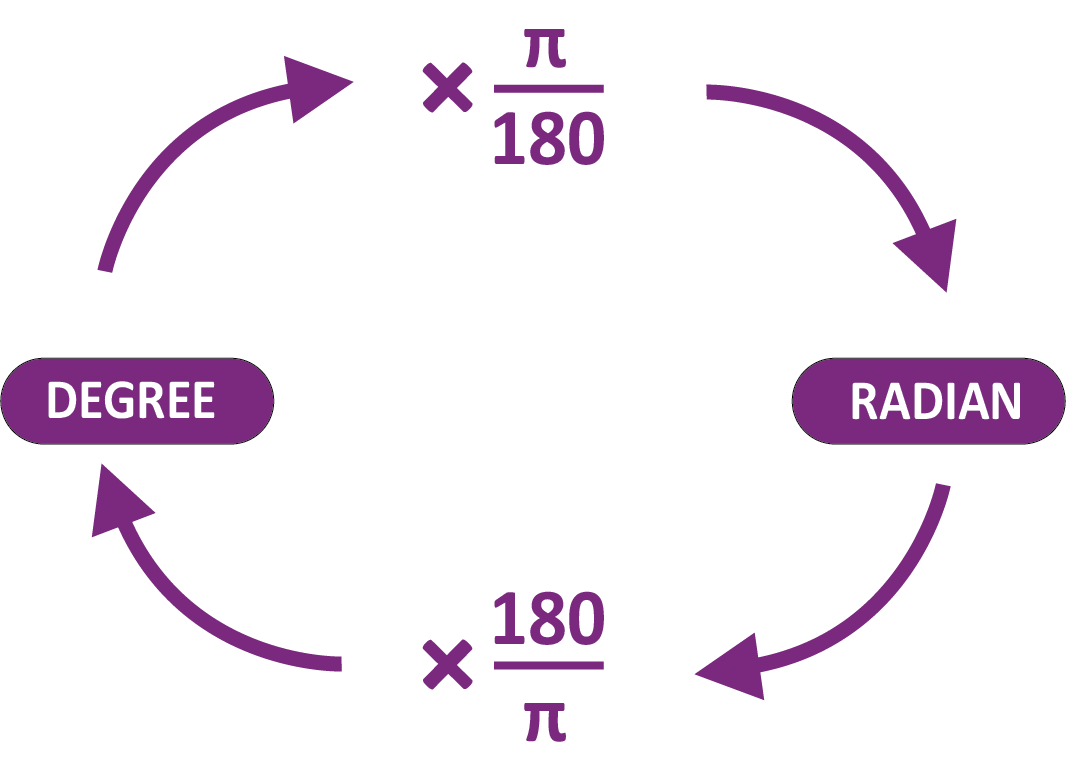Convert

The relation between degree measures and radian measures of some standard angles can be observed.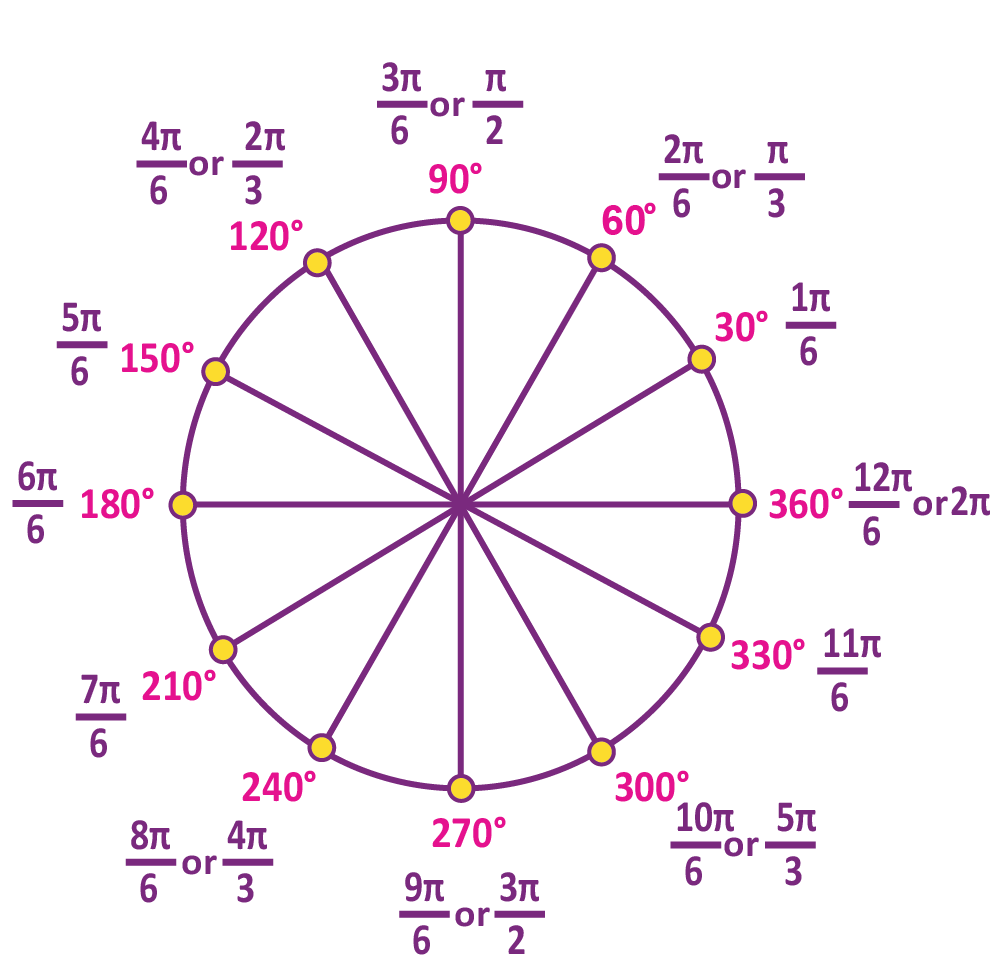Example 1: Convert 135 degrees to radians.

Solution:

1350 = 1350 x (π/1800)

Or

### Table for Degree and Radian Relation:

 Degree 300 450 600 900 1800 2700 3600 Radian π/6 π/4 π/3 π/2 π 3π/2 2π

### Trigonometry Table:

 Angle 0 π/6 π/4 π/3 π/2 π 3π/2 2π $\operatorname{Sin} \theta$ 0 1/2 1/√2 √3/2 1 0 -1 0 $\operatorname{Cos} \theta$ 1 √3/2 1/√2 1/2 0 -1 0 1 $\operatorname{Tan} \theta$ 0 1/√3 1 √3 undefined 0 undefined 0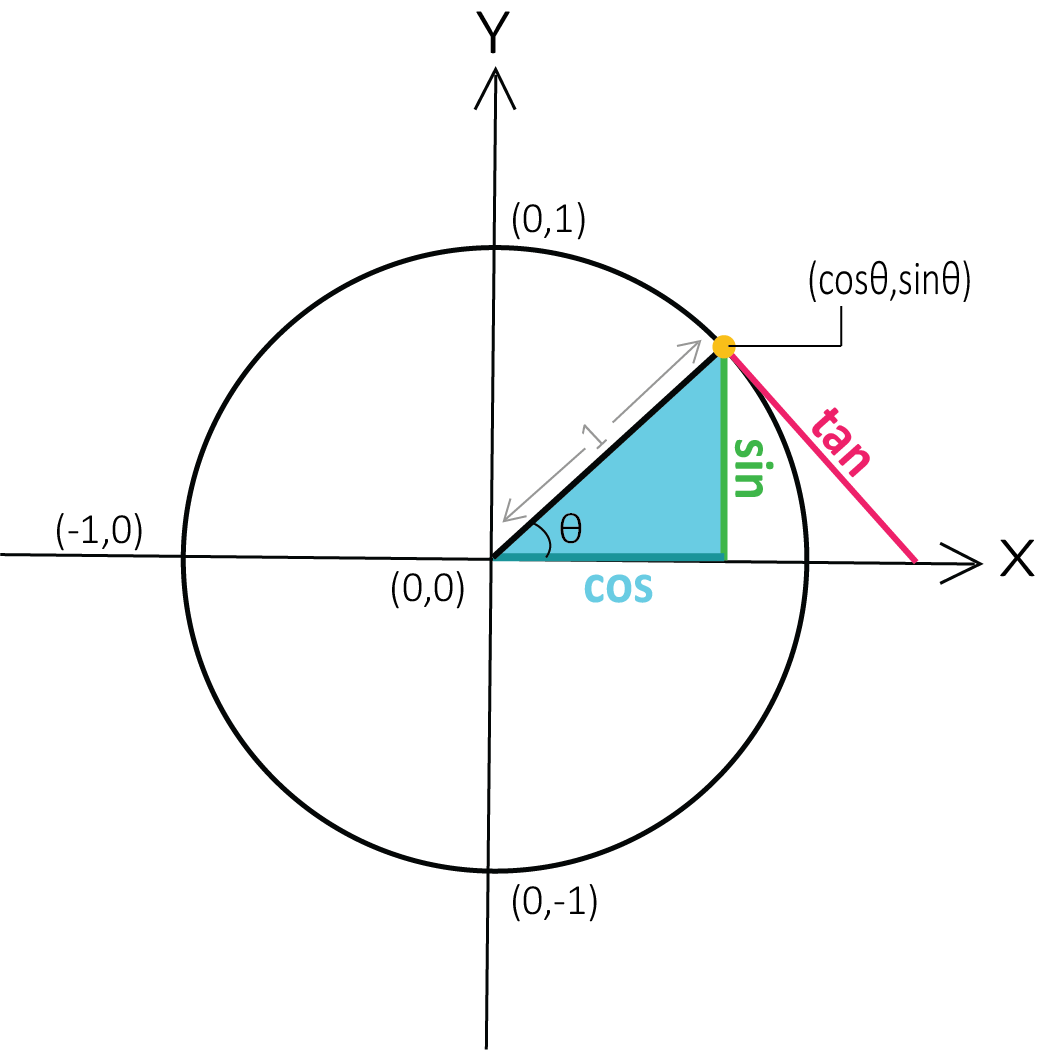Trigonometric Signs

Sign of Trigonometry Functions:

$(\operatorname{Cos} {( - }\theta ) = \operatorname{Cos} \theta$

$\operatorname{Sin} {(- }\theta ){ = - }\operatorname{Sin} \theta$

$\operatorname{Tan} {(- }\theta ){ = - }Tan~\theta$

$Cot{(^ - }\theta ){ = - }Cot~\theta$

$\operatorname{Sec} {(- }\theta ) = \operatorname{Sec} \theta$

$\operatorname{csc} {( - }\theta ){ = - }\operatorname{csc}\theta$

In trigonometry, there are six functions, namely sin, cos, tan, csc, sec and tan. We can determine the sign of the trigonometric function with the help of a unit circle. Let P(a, b) be a point on the unit circle with the centre at the origin.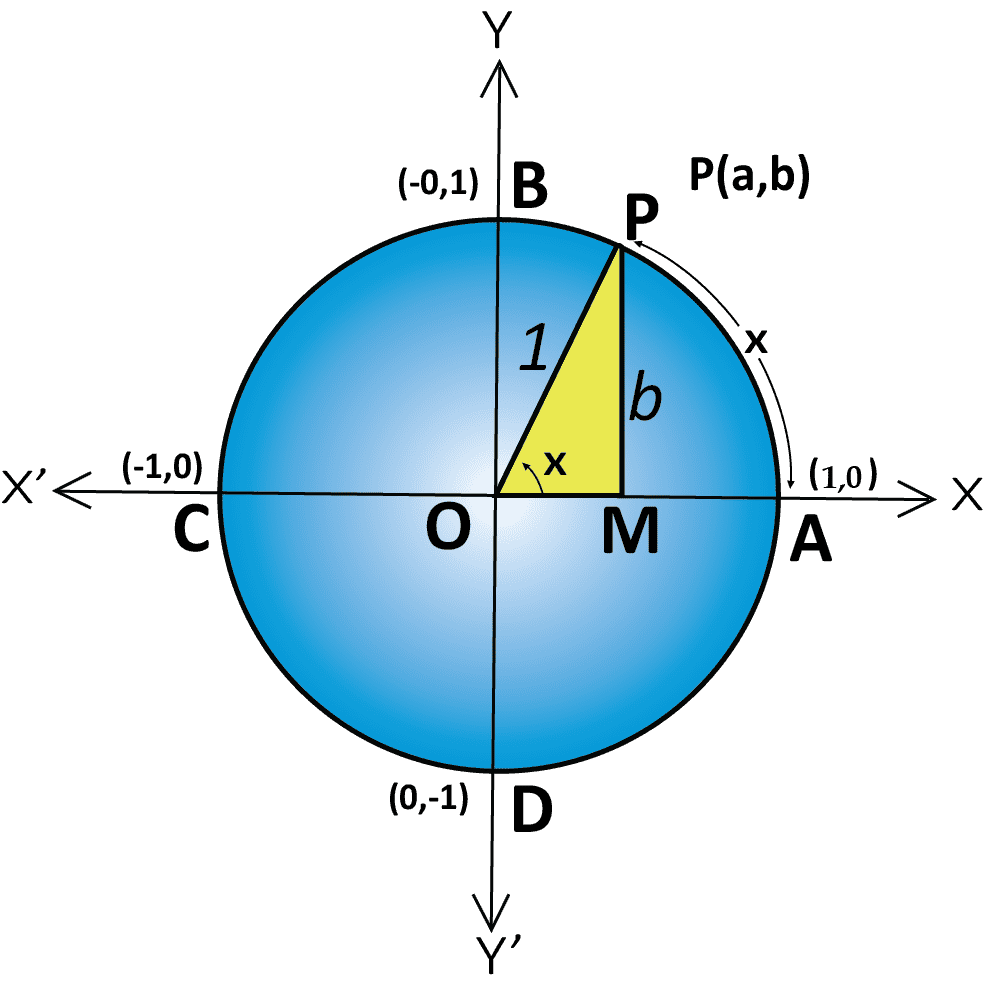Function of Trigonometry

For every point P (a, b) on the unit circle, – 1 ≤ a ≤ 1 and – 1 ≤ b ≤ 1, we have – 1 ≤ cos x ≤ 1 and –1 ≤ sin x ≤ 1 for all x. Also, we can define the values of a and b in different quadrants as

The first quadrant: 0 < x < π/2 ⇒ a and b are positive

Second quadrant: π/2 < x < π ⇒ a is negative and b is positive

Third quadrant: π < x < 3π/2 ⇒ a and b are negative

Fourth quadrant: 3π/2 < x < 2π ⇒ a is positive and b is negative.

### The Sign of Trigonometric Functions in Each Quadrant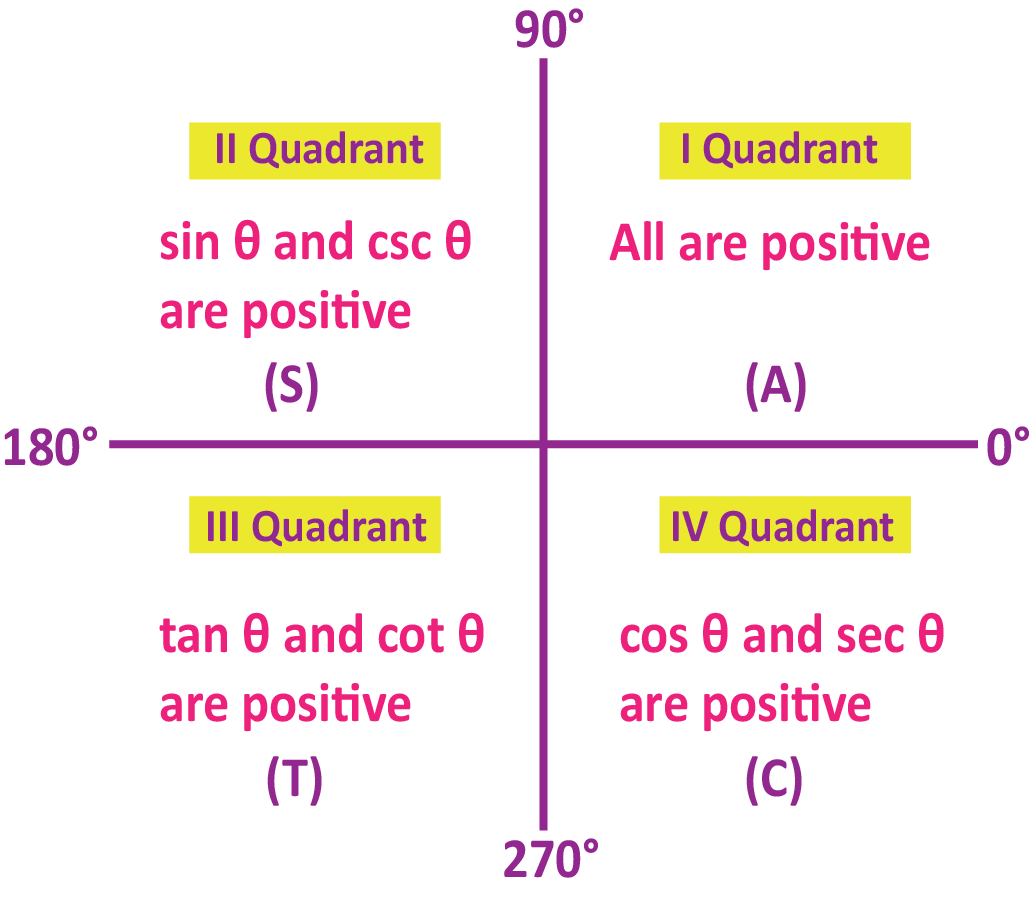In the first quadrant, all functions are positive, in the 2nd quadrant, only sin and csc are positive, in the 3rd quadrant only tan and cot are positive, whereas in the 4th quadrant only cos and sec are positive. Thus, we can tabulate the signs of different trigonometric functions in different quadrants as follows:

 Quadrant I Quadrant II Quadrant III Quadrant IV sin θ +ve +ve -ve -ve cos θ +ve -ve -ve +ve tan θ +ve -ve +ve -ve csc θ +ve +ve -ve -ve sec θ +ve -ve -ve +ve cot θ +ve -ve +ve -ve

Also, go through the table given below to understand the behaviour of trigonometric functions with respect to their values in different quadrants.

 Quadrant I Quadrant II Quadrant III Quadrant IV sin Increases from 0 to 1 Decreases from 1 to 0 Decreases from o to -1 Increases from -1 to 0 cos Decreases from 1 to 0 Decreases from o to -1 Increases from -1 to 0 Increases from 0 to 1 tan Increases from 0 to $\infty$ Increases from -$\infty$ to 0 Increases from 0 to $\infty$ Increases from -$\infty$ to 0 csc Decreases from $\infty$ to 1 Increases from 1 to $\infty$ Increases from -$\infty$ to 1 Decreases from -1 to $\infty$ sec Increases from 1 to $\infty$ Increases from -$\infty$ to 1 Decreases from -1 to $\infty$ Decreases from $\infty$ to 1 cot Decreases from $\infty$ to 0 Decreases from 0 to -$\infty$ Decreases from $\infty$ to 0 Decreases from 0 to -$\infty$

### Graphs of Trigonometry Functions:

The trigonometric functions are:

1. Sine

2. Cosine

3. Tangent

4. cosec

5. Secant

6. Cotangent

Sine, Cosine and tangent are the three important trigonometry ratios, based on which functions are defined. Below are the graphs of the three trigonometry functions sin x, cos x, and tan x. In these trigonometry graphs, x-axis values of the angles are in radians, and on the y-axis, its f(x) is taken, the value of the function at each given angle.

### Sin Graph:

• $Y = \sin x$

• The roots or zeros of $Y = \sin x$ is at the multiples of π

• The sin graph passes the x-axis as sin x = 0 there

• Period of the sine function is

• The height of the curve at each point is equal to the line value of sine

 Max value of Graph Min value of the graph 1 at  π/2 -1  at  (3π/2)

### Cos Graph:

• $Y = \cos x$

• $\sin \left( {x + \dfrac{\pi }{2}} \right)$ = cos x

• y = cos x graph is the graph we get after shifting $Y = \sin x$ to  π/2 units to the left

• Period of the cosine function is

 Max value of Graph Min value of the graph 1 at 0, 4π -1  at 2π

There are a few similarities between the sine and cosine graphs, They are:

1. Both have the same curve which is shifted along the x-axis

2. Both have an amplitude of 1

3. Have a period of 360° or 2π radians

The combined graph of sine and cosine function can be represented as follows.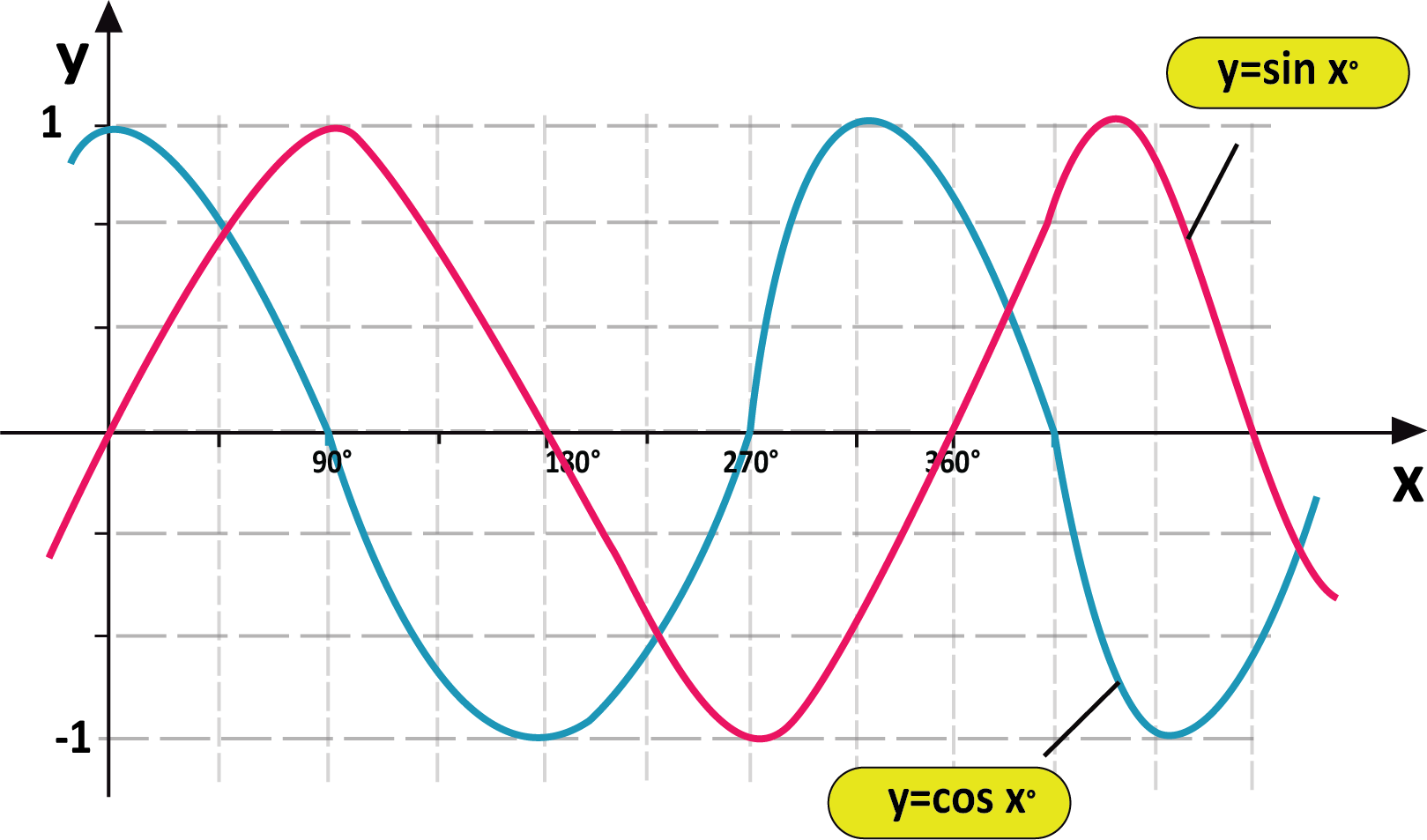Tan Graph

Example: If $\cot x = - \dfrac{5}{{12}}$, x lies in the fourth quadrant, then find the value of csc x.

Solution: Given, $\cot x = - \dfrac{5}{{12}}$

We know that,

$\csc{^2}x - {\cot ^2}x = 1$

$\csc{^2}x = 1 + {\cot ^2}x$

= $1 + {\left( {\dfrac{{ - 5}}{{12}}} \right)^2}$

= $1 + \left( {\dfrac{{25}}{{144}}} \right)$

= $1 + \dfrac{{\left( {144 + 25} \right)}}{{144}}$

= $\dfrac{{169}}{{144}}$

$\csc~x = \sqrt {\left( {\dfrac{{169}}{{144}}} \right)} = \pm \dfrac{{13}}{{12}}$

Given that x lies in the fourth quadrant and csc x is negative in the fourth quadrant.

Therefore, $\csc~x = - \dfrac{{13}}{{12}}$

Similarly, we can solve various types of trigonometry problems quickly. These are useful in determining the values of trigonometry functions which are dependent on other functions and trigonometry angles also.

Example: What will be the value of $\sec ^{-1}\left(\dfrac{1}{4} \sum_{k=0}^{10} \sec \left(\dfrac{7 \pi}{12}+\dfrac{k \pi}{2}\right) \sec \left(\dfrac{7 \pi}{12}+\dfrac{(k+1) \pi}{2}\right)\right)$ in the interval $\left[-\dfrac{\pi}{4}, \dfrac{3 \pi}{4}\right]$ equals to _________.

Explanation

$\sec ^{-1}\left(\dfrac{1}{4} \sum_{k=0}^{10} \sec \left(\dfrac{7 \pi}{12}+\dfrac{k \pi}{2}\right) \sec \left(\dfrac{7 \pi}{12}+\dfrac{(k+1) \pi}{2}\right)\right)$

$=\sec ^{-1}\left(-\dfrac{1}{4} \sum_{k=0}^{10} \sec \left(\dfrac{7 \pi}{12}+\dfrac{k \pi}{2}\right) \operatorname{csc}\left(\dfrac{7 \pi}{12}+\dfrac{k \pi}{2}\right)\right)$

$=\sec ^{-1}\left(-\dfrac{1}{2} \sum_{k=0}^{10} \dfrac{1}{\sin \left(\dfrac{7 \pi}{6}\right) \cdot(-1)^{k}}\right)$

$=\sec ^{-1}\left(-\dfrac{1}{2} \dfrac{1}{\sin \left(\dfrac{7 \pi}{6}\right)}\right)$

$=\sec ^{-1}(1)=0$

## Importance of Trigonometry

Trigonometric ratios are determined by measuring the length of different sides of a right-angled triangle. On proceeding further, the relationships between two trigonometric ratios can also be identified using various formulas.

These relationships become universal for all kinds of angles existing in a right-angled triangle. Hence, these relationships are then termed identities. They will remain the same and can be used for solving sums based on trigonometry.

The trigonometric functions are covered in this chapter along with some changes in values and signs. Learn how these functions are applied to different cases. This chapter is of utmost importance as the knowledge of trigonometric functions will be required in different fields as well.

This chapter helps students lay a strong foundation for the values of trigonometric ratios of various angles. These revision notes will help them determine the implications of such functions and identities easily.

## Benefits of Trigonometry JEE Advanced Revision Notes

• These revision notes have been prepared by the top teachers of Vedantu providing an easier explanation of all the identities and formulas covered in this chapter. The basic concept of formulating a trigonometric identity or a function will become much clearer to you with the help of these notes.

• Use these notes to prepare and revise this chapter before the exams. There is no need to make notes by yourself when you can avail of these expert-prepared notes for free.

• Refer to these revision notes to solve and practice the trigonometric identities and functions at your convenience and to study this chapter efficiently.

• Solve and practice the sums of this chapter with the help of these revision notes to evaluate your understanding. Thus, you can find out the areas in which you need to work harder and complete your Trigonometry JEE Advanced revision before the exam.

You can download these revision notes in PDF format and make a quick review of the concepts covered in this chapter. Going through these notes will help you to remember all you have studied in this chapter. Find out how the experts have solved sample questions and learn the techniques to determine the right answers from these revision notes. Trigonometry can be a little tricky as the same identities have multiple applications. So refer to this Trigonometry JEE Advanced Notes PDF for your exam preparation and score well in the exam.

## FAQs on JEE Advanced 2023 Revision Notes for Trigonometry

1. What is a trigonometric ratio?

The ratio of the length of two sides constituting an angle in a right-angled triangle is called a trigonometric ratio. The length of the sides will depend on the ratio.

2. Why should I remember the value of trigonometric ratios of some common angles?

The values of trigonometric ratios of some common angles will help you identify the value of trigonometric equations faster. You can accurately find the answers if you learn the values of some common trigonometric ratios.

3. What is a right-angled triangle?

A triangle with one of its three interior angles measuring 90-degree is called a right-angled triangle.

4. What is the application of trigonometric ratios?

Using trigonometric ratios, you can determine the height and distance between two objects like buildings, towers, etc.